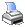Navigation:  Reference > Structured Programming Commands > IF/ENDIFThis is a non-loop structure.  Each item is only tested once.  The general layout of the structure is:

IF lexpr

ELSE_IF lexpr

ELSE

ENDIF

 IF lexpr This is the beginning of the IF/ENDIF structure.  The lexpr must be in the form of a comparison expression.  For example:

IF x = 1

If the lexpr returns .TRUE., the lines after the IF command and continuing until the next ELSE_IF, ELSE or ENDIF command will be executed.  Otherwise control will be passed to the next subsequent ELSE_IF, ELSE, or ENDIF command.

ELSE_IF lexpr  If the original IF or a previous ELSE_IF comparison expression returned .FALSE., the program will keep testing each subsequent ELSE_IF command until one is found that returns .TRUE. or until an ELSE command is found, or the appropriate ENDIF command is found.  If the ELSE_IF lexpr returns .T., the lines after the command will be executed until the next ELSE_IF, ELSE, or ENDIF command.

There can be any number of ELSE_IF commands within an IF/ENDIF structure.  However, only one will be executed, and that will be the first one for which the lexpr resolves to .TRUE.  Once the program lines have been executed, control is transferred to the appropriate ENDIF command.

 ELSE If the original IF and the previous ELSE_IF commands all resolved to .FALSE., the program will always execute the lines after this command and until the ENDIF.  This is a way of providing for actions to be taken if all the other IF tests have failed.

 ENDIF This is the end of the IF/ENDIF structure.  Each IF must have an ENDIF to finish the structure.  If the ENDIF is not included an error message will be displayed during compilation.

EXAMPLE

The following example shows the proper way to use this structure:

IF X = 100

...

...        && group 1

...

ELSE_IF X = 200

...

...        && group 2

...

ELSE_IF X = 300

...

...        && group 3

...

ELSE

...

...        && group 4

...

ENDIF

(The indentations are for clarity only.)  In the example above if x = 100 the lines in group 1 will be executed, if x = 200 group 2, and if x = 300 group 3.  Otherwise the command lines in group 4 will be executed.